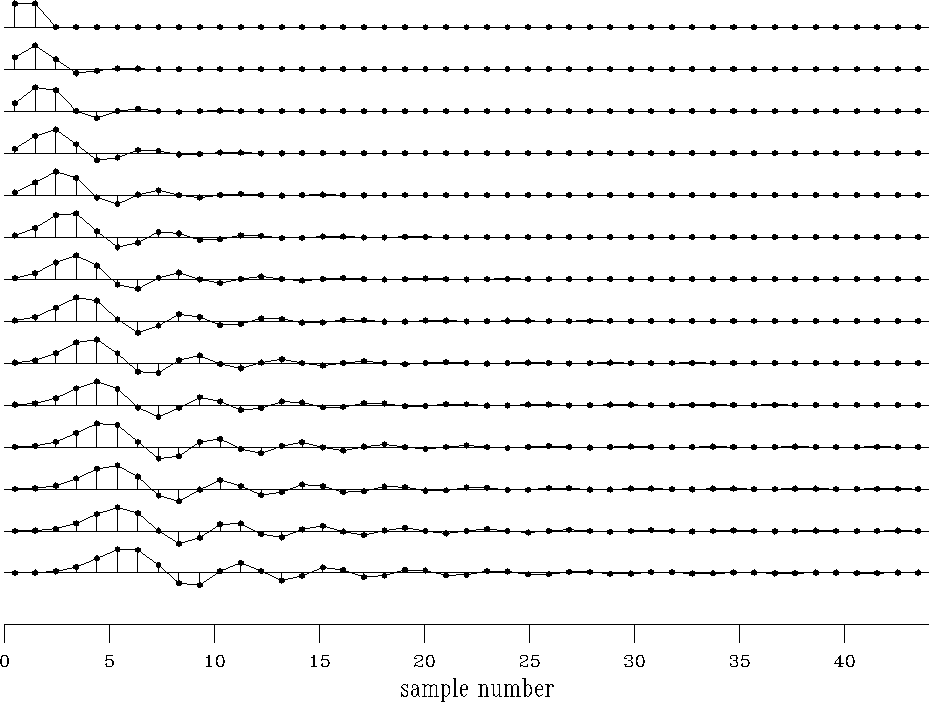Next: PHASE DELAY AND GROUP Up: A BUTTERWORTH-FILTER COOKBOOK Previous: Butterworth-filter finding program

## Examples of Butterworth filters

Spectra and log spectra of various orders of Butterworth filters are shown in Figure 14. They match a rectangle function that passes frequencies below the half-Nyquist. Convergence is rapid with order. The logarithm plot shows a range of 0-3, meaning an amplitude ratio of 103=1000. Tiny glitches near the bottom for high-order curves result from truncating the time axis in the time domain shown in Figure 15.

 butm Figure 15 Butterworth-filter time responses for half-Nyquist low pass.The time-domain truncation also explains a slight roughness on the top of the rectangle function.

In practice, the filter is sometimes run both forward and backward to achieve a phaseless symmetrical response. This squares the spectral amplitudes, resulting in convergence twice as fast as shown in the figure. Notice that the higher-order curves in the time domain (Figure 15) have undesirable sidelobes which ring longer with higher orders. Also, higher-order curves have increasing delay for the main signal burst. This delay is a consequence of the binomial coefficients in the numerator.

Another example of a low-pass Butterworth filter shows some lurking instability. This is not surprising: a causal bandpass operator is almost a contradiction in terms, since the word ``bandpass'' implies multiplying the spectrum by zero outside the chosen band, and the word ``causal'' implies a well-behaved spectral logarithm.

These cannot coexist because the logarithm of zero is minus infinity. All this is another way of saying that when we use Butterworth filters, we probably should not use high orders. Figure 16 illustrates that an instability arises in the seventh-order Butterworth filter, and even the sixth-order filter looks suspicious.

 butl Figure 16 Butterworth time responses for a narrow-band low-pass filter.If we insist on using high-order filters, we can probably go to an order about twice as high as we began with by using double precision, increasing the spectral width nw, and, if we are really persistent, using the method of the exercises below. My favorite Butterworth filters for making synthetic seismograms have five coefficients (fourth order). I do one pass through a low cut at cutoff=.1 and another through a high cut at cutoff=.4.

## EXERCISES:

1. Above we assumed that a bandpass filter should be made by cascading a low-pass and a high-pass filter. Suggest a revised form of equation (21) for making bandpass filters directly.
2. Notice that equation (21) can be factored analytically. Individual factors could be implemented as the Z-transform filters, and the filters cascaded. This prevents the instability that arises when many poles are combined. Identify the poles of equation (21). Which belong in the causal filter and which in its time reverse?Next: PHASE DELAY AND GROUP Up: A BUTTERWORTH-FILTER COOKBOOK Previous: Butterworth-filter finding program
Stanford Exploration Project
10/21/1998